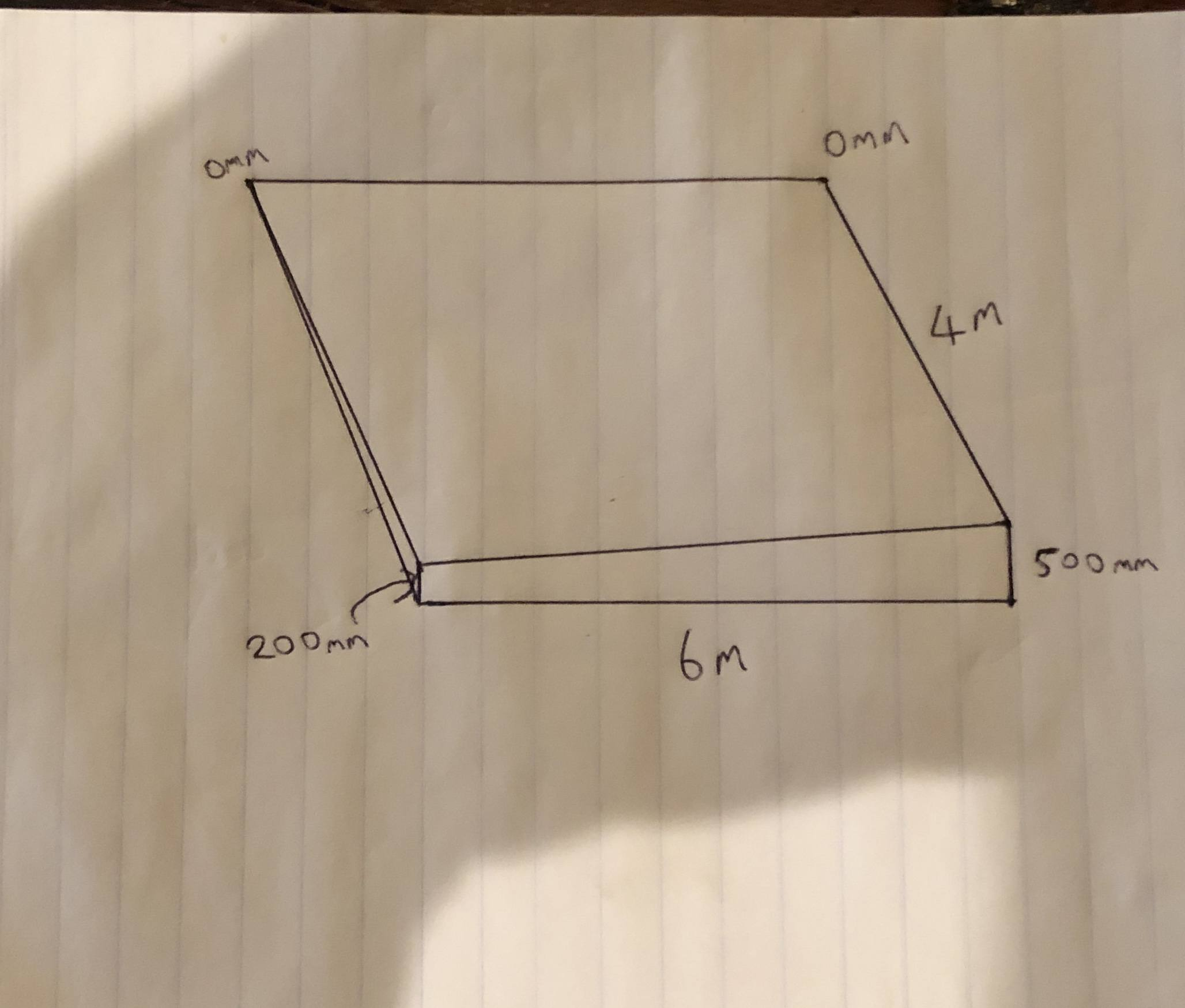# Calculate volume of non parallel wedge

• MHB
• jasonmcbride

#### jasonmcbride

Hi, just wondering how I would calculate the volume of this wedge? see photo attachedWe have a triangle on the left side with area $\frac 12\cdot 4\,\text{m}\cdot 200\,\text{mm} = 0.4\,\text{m}^2$.
And we have a triangle on the right side with area $\frac 12\cdot 4\,\text{m}\cdot 500\,\text{mm} = 1.0\,\text{m}^2$.
The average triangular area is $\frac{0.4+1.0}{2} = 0.7\,\text{m}^2$.
Multiply by the length to find the volume $V=6\,\text{m}\cdot 0.7\,\text{m}^2=4.2\,\text{m}^3$.

Btw, the top surface is not flat.

Last edited: